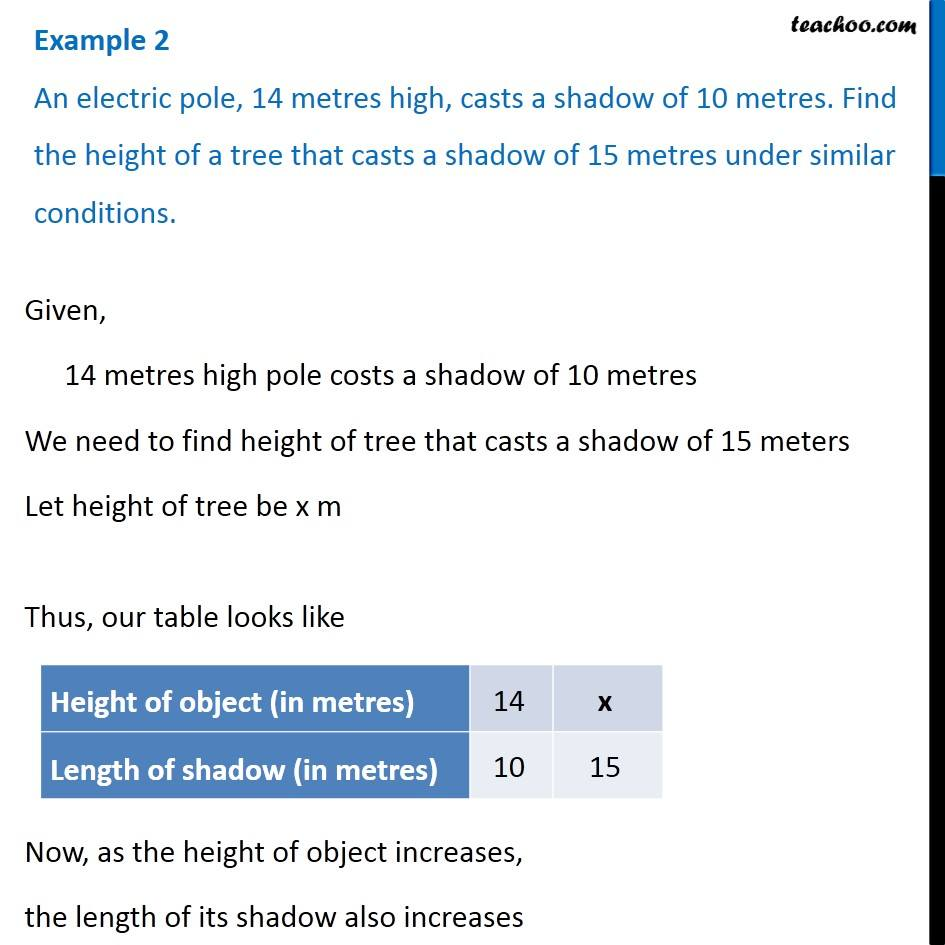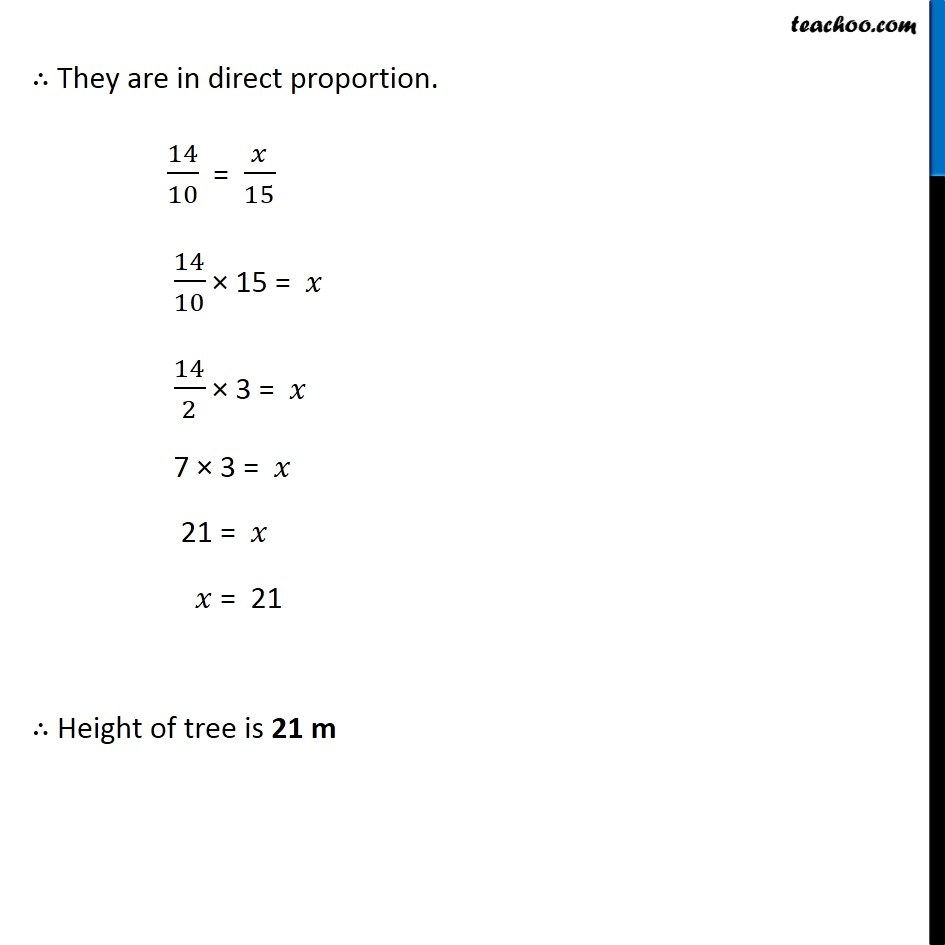1. Chapter 13 Class 8 Direct and Inverse Proportions
2. Concept wise
3. Direct Proportion

Transcript

Example 2 An electric pole, 14 metres high, casts a shadow of 10 metres. Find the height of a tree that casts a shadow of 15 metres under similar conditions. Given, 14 metres high pole costs a shadow of 10 metres We need to find height of tree that casts a shadow of 15 meters Let height of tree be x m Thus, our table looks like Now, as the height of object increases, the length of its shadow also increases ∴ They are in direct proportion. 14/10 = 𝑥/15 14/10 × 15 = 𝑥 14/2 × 3 = 𝑥 7 × 3 = 𝑥 21 = 𝑥 𝑥 = 21 ∴ Height of tree is 21 m

Direct Proportion